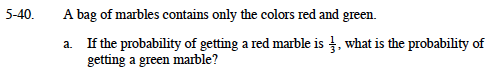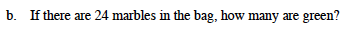### Home > MC1 > Chapter 5 > Lesson 5.2.1 > Problem5-40

5-40.The probability stated means that if there are 3 marbles in the bag, there is only one chance that you will pull out a red marble. How many chances are there that you will pull out a green marble?

If there were only three marbles in the bag, and one of them is red, then the other 2 must be green. This means that 2 out of 3 marbles are green, so the probability must be

$\frac{2}{3}.$

Another way to think of this is that if 1 of every 3 marbles is red, then the other 2 of every 3 must be green.If the probability of picking a green marble is two-thirds, that means two-thirds of the marbles are green. What is two-thirds of 24?

How could you represent two-thirds in a fraction with the denominator, or bottom number, of 24? The numerator, or number on top, will be the number of green marbles.